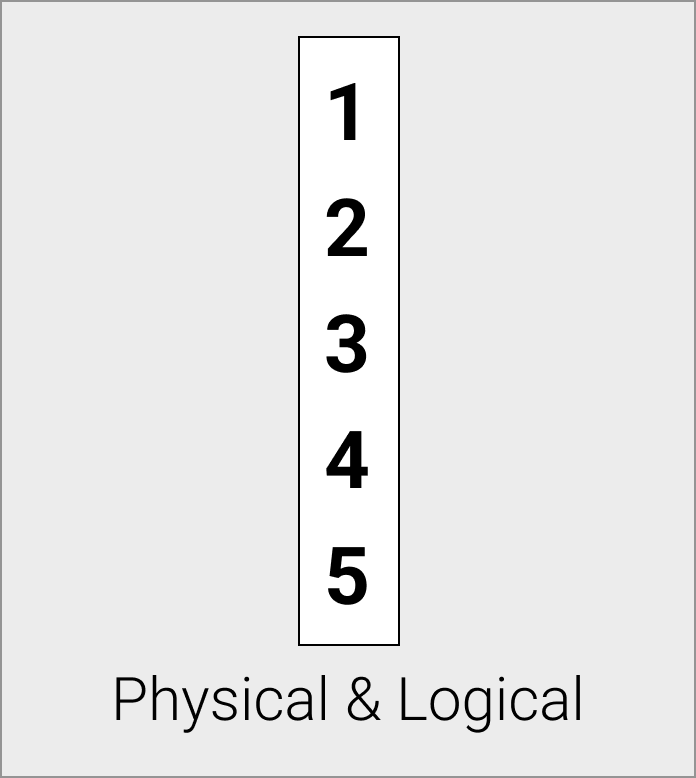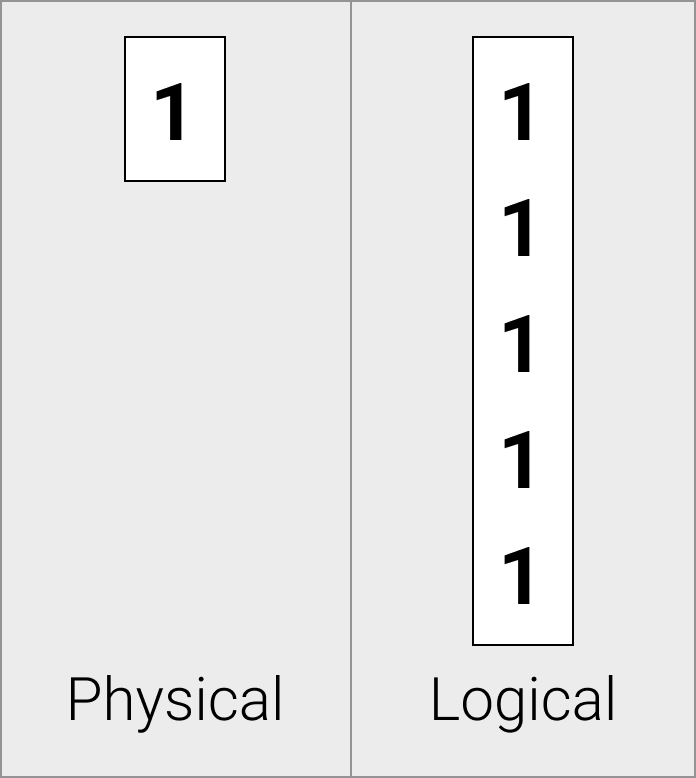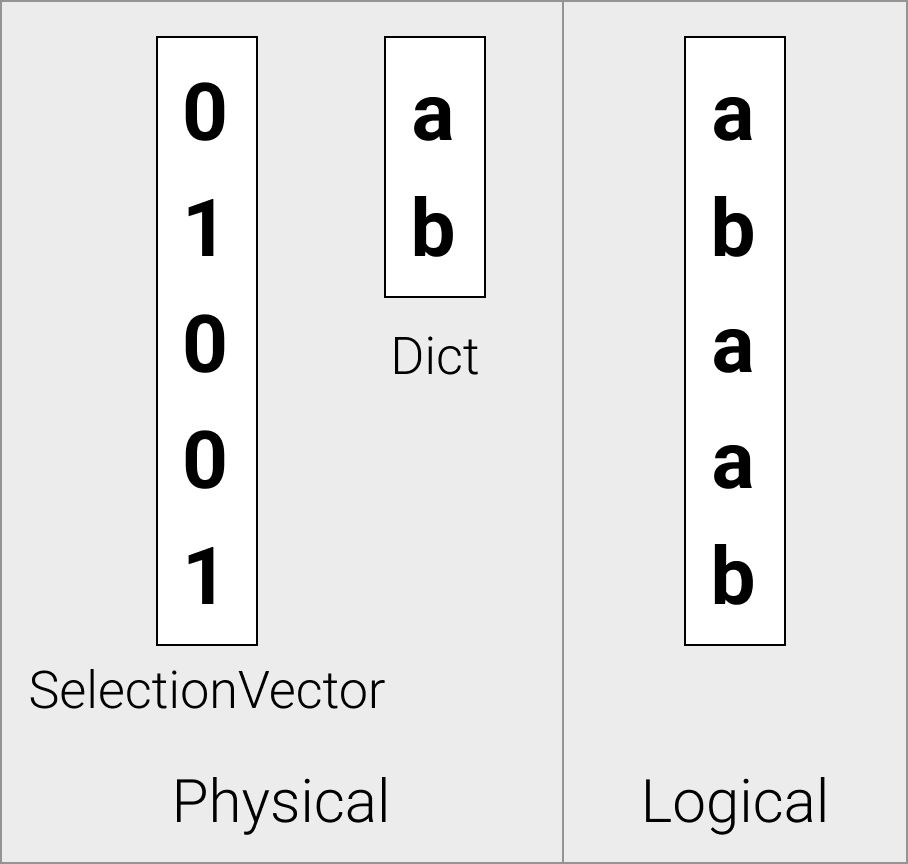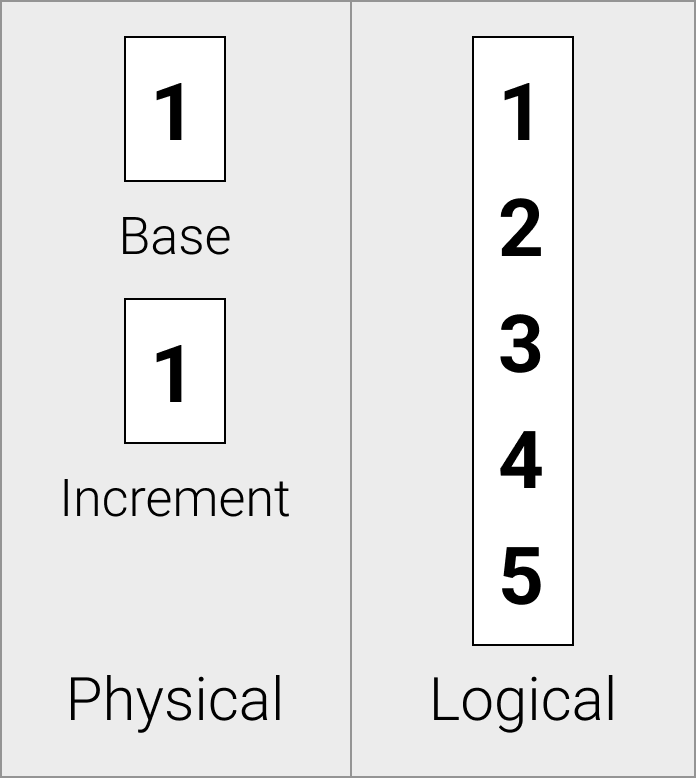Execution Format

`Vector` is the container format used to store in-memory data during execution.
`DataChunk` is a collection of Vectors, used for instance to represent a column list in a PhysicalProjection operator.

## Data Flow

DuckDB uses a vectorized query execution model.
All operators in DuckDB are optimized to work on Vectors of a fixed size.

This fixed size is commonly referred to in the code as `STANDARD_VECTOR_SIZE`.
The default `STANDARD_VECTOR_SIZE` is 2048 tuples.

## Vector Format

Vectors logically represent arrays that contain data of a single type. DuckDB supports different vector formats, which allow the system to store the same logical data with a different physical representation. This allows for a more compressed representation, and potentially allows for compressed execution throughout the system. Below the list of supported vector formats is shown.

### Flat Vectors

Flat vectors are physically stored as a contiguous array, this is the standard uncompressed vector format. For flat vectors the logical and physical representations are identical.### Constant Vectors

Constant vectors are physically stored as a single constant value.Constant vectors are useful when data elements are repeated - for example, when representing the result of a constant expression in a function call, the constant vector allows us to only store the value once.

``````select lst || 'duckdb' from range(1000) tbl(lst);
``````

Since `duckdb` is a string literal, the value of the literal is the same for every row. In a flat vector, we would have to duplicate the literal ‘duckdb’ once for every row. The constant vector allows us to only store the literal once.

Constant vectors are also emitted by the storage when decompressing from constant compression.

### Dictionary Vectors

Dictionary vectors are physically stored as a child vector, and a selection vector that contains indices into the child vector.Dictionary vectors are emitted by the storage when decompressing from dictionary

Just like constant vectors, dictionary vectors are also emitted by the storage.
When deserializing a dictionary compressed column segment, we store this in a dictionary vector so we can keep the data compressed during query execution.

### Sequence Vectors

Sequence vectors are physically stored as an offset and an increment value.Sequence vectors are useful for efficiently storing incremental sequences. They are generally emitted for row identifiers.

### Unified Vector Format

These properties of the different vector formats are great for optimization purposes, for example you can imagine the scenario where all the parameters to a function are constant, we can just compute the result once and emit a constant vector.
But writing specialized code for every combination of vector types for every function is unfeasible due to the combinatorial explosion of possibilities.

Instead of doing this, whenever you want to generically use a vector regardless of the type, the UnifiedVectorFormat can be used.
This format essentially acts as a generic view over the contents of the Vector. Every type of Vector can convert to this format.

## Complex Types

### String Vectors

To efficiently store strings, we make use of our `string_t` class.

``````struct string_t {
union {
struct {
uint32_t length;
char prefix;
char *ptr;
} pointer;
struct {
uint32_t length;
char inlined;
} inlined;
} value;
};
``````

Short strings (`<= 12 bytes`) are inlined into the structure, while larger strings are stored with a pointer to the data in the auxiliary string buffer. The length is used throughout the functions to avoid having to call `strlen` and having to continuously check for null-pointers. The prefix is used for comparisons as an early out (when the prefix does not match, we know the strings are not equal and don’t need to chase any pointers).

### List Vectors

List vectors are stored as a series of list entries together with a child Vector. The child vector contains the values that are present in the list, and the list entries specify how each individual list is constructed.

``````struct list_entry_t {
idx_t offset;
idx_t length;
};
``````

The offset refers to the start row in the child Vector, the length keeps track of the size of the list of this row.

List vectors can be stored recursively. For nested list vectors, the child of a list vector is again a list vector.

For example, consider this mock representation of a Vector of type `BIGINT[][]`:

``````{
"type": "list",
"data": "list_entry_t",
"child": {
"type": "list",
"data": "list_entry_t",
"child": {
"type": "bigint",
"data": "int64_t"
}
}
}
``````

### Struct Vectors

Struct vectors store a list of child vectors. The number and types of the child vectors is defined by the schema of the struct.

### Map Vectors

Internally map vectors are stored as a `LIST[STRUCT(key KEY_TYPE, value VALUE_TYPE)]`.

### Union Vectors

Internally `UNION` utilizes the same structure as a `STRUCT`. The first “child” is always occupied by the Tag Vector of the UNION, which records for each row which of the UNION’s types apply to that row.

Search Shortcut cmd + k | ctrl + k